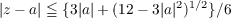#### Vol. 41, No. 2, 1972

 Download this articleFor screen For printingRecent Issues Vol. 325: 1 Vol. 324: 1  2 Vol. 323: 1  2 Vol. 322: 1  2 Vol. 321: 1  2 Vol. 320: 1  2 Vol. 319: 1  2 Vol. 318: 1  2Online Archive Volume: Issue:The Journal Subscriptions Editorial Board Officers Contacts Submission Guidelines Submission Form Policies for Authors ISSN: 1945-5844 (e-only) ISSN: 0030-8730 (print) Special Issues Author Index To Appear Other MSP Journals
On the zeros of a polynomial and its derivative

Vol. 41 (1972), No. 2, 525–528
##### Abstract

Let all the zeros of a polynomial p(z) of degree n lie in |z|1. Given a complex number a what is the radius of the smallest disk centred at α containing at least one zero of the polynomial ((z a)p(z))f? According to Theorem 1 the answer is (|a| + 1)(n + 1) if |α| > (n + 2)∕n. Theorem 2 which states that if both the zeros of the quadratic polynomial p(z) lie in |z|1 and |a|2 then ((z α)p(z))has at least one zero incompletely settles the case n = 2.

Primary: 30A08
##### Milestones